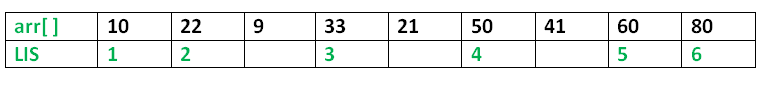# C++ Program for Longest Increasing Subsequence

The Longest Increasing Subsequence (LIS) problem is to find the length of the longest subsequence of a given sequence such that all elements of the subsequence are sorted in increasing order. For example, the length of LIS for {10, 22, 9, 33, 21, 50, 41, 60, 80} is 6 and LIS is {10, 22, 33, 50, 60, 80}.Examples:

```Input  : arr[] = {3, 10, 2, 1, 20}
Output : Length of LIS = 3
The longest increasing subsequence is 3, 10, 20

Input  : arr[] = {3, 2}
Output : Length of LIS = 1
The longest increasing subsequences are {3} and {2}

Input : arr[] = {50, 3, 10, 7, 40, 80}
Output : Length of LIS = 4
The longest increasing subsequence is {3, 7, 40, 80}
```

Overlapping Subproblems:
Considering the above implementation, following is recursion tree for an array of size 4. lis(n) gives us the length of LIS for arr[].

```              lis(4)
/        |
lis(3)    lis(2)   lis(1)
/           /
lis(2) lis(1) lis(1)
/
lis(1)
```

We can see that there are many subproblems which are solved again and again. So this problem has Overlapping Substructure property and recomputation of same subproblems can be avoided by either using Memoization or Tabulation. Following is a tabulated implementation for the LIS problem.

## C++

 `/* Dynamic Programming C/C++ implementation of LIS problem */` `#include ` `#include ` ` `  `/* lis() returns the length of the longest increasing ` `  ``subsequence in arr[] of size n */` `int` `lis(``int` `arr[], ``int` `n) ` `{ ` `    ``int` `*lis, i, j, max = 0; ` `    ``lis = (``int``*)``malloc``(``sizeof``(``int``) * n); ` ` `  `    ``/* Initialize LIS values for all indexes */` `    ``for` `(i = 0; i < n; i++) ` `        ``lis[i] = 1; ` ` `  `    ``/* Compute optimized LIS values in bottom up manner */` `    ``for` `(i = 1; i < n; i++) ` `        ``for` `(j = 0; j < i; j++) ` `            ``if` `(arr[i] > arr[j] && lis[i] < lis[j] + 1) ` `                ``lis[i] = lis[j] + 1; ` ` `  `    ``/* Pick maximum of all LIS values */` `    ``for` `(i = 0; i < n; i++) ` `        ``if` `(max < lis[i]) ` `            ``max = lis[i]; ` ` `  `    ``/* Free memory to avoid memory leak */` `    ``free``(lis); ` ` `  `    ``return` `max; ` `} ` ` `  `/* Driver program to test above function */` `int` `main() ` `{ ` `    ``int` `arr[] = { 10, 22, 9, 33, 21, 50, 41, 60 }; ` `    ``int` `n = ``sizeof``(arr) / ``sizeof``(arr); ` `    ``printf``(``"Length of lis is %d\n"``, lis(arr, n)); ` `    ``return` `0; ` `} `

## Java

 `/* Dynamic Programming Java implementation ` `of LIS problem */` `import` `java.util.*; ` ` `  `class` `GFG  ` `{ ` ` `  `    ``/* ` `    ``* lis() returns the length of the longest  ` `    ``* increasing subsequence in arr[] of size n ` `    ``*/` `    ``static` `int` `lis(``int``[] arr, ``int` `n)  ` `    ``{ ` `        ``int` `max = ``0``; ` `        ``int``[] lst = ``new` `int``[n]; ` ` `  `        ``// initialize LIS values for all indexes ` `        ``Arrays.fill(lst, ``1``); ` ` `  `        ``/* Compute optimized LIS values  ` `        ``in bottom up manner */` `        ``for` `(``int` `i = ``1``; i < n; i++)  ` `        ``{ ` `            ``for` `(``int` `j = ``0``; j < i; j++)  ` `            ``{ ` `                ``if` `(arr[i] > arr[j] &&  ` `                    ``lst[i] < lst[j] + ``1``) ` `                    ``lst[i] = lst[j] + ``1``; ` `            ``} ` `        ``} ` ` `  `        ``/* Pick maximum of all LIS values */` `        ``for` `(``int` `i = ``0``; i < n; i++) ` `            ``if` `(max < lst[i]) ` `                ``max = lst[i]; ` ` `  `        ``return` `max; ` `    ``} ` ` `  `    ``// Driver Code ` `    ``public` `static` `void` `main(String[] args)  ` `    ``{ ` `        ``int``[] arr = { ``10``, ``22``, ``9``, ``33``, ``21``, ``50``, ``41``, ``60` `}; ` `        ``int` `n = arr.length; ` `        ``System.out.println(``"Length of lis is "` `+  ` `                                   ``lis(arr, n)); ` `    ``} ` `} ` ` `  `// This code is contributed by ` `// sanjeev2552 `

## Python3

 `# Dyanmic Programming python3 ` `# implementation of LIS problem ` ` `  `# lis() returns the length of the  ` `# longest increasing subsequence  ` `# in arr[] of size n ` `def` `lis(arr, n): ` `    ``i, j, maxm ``=` `0``, ``0``, ``0` `     `  `    ``# initialize LIS values for all indexes ` `    ``lst ``=` `[``1` `for` `s ``in` `range``(n)] ` `     `  `    ``for` `i ``in` `range``(``1``, n): ` `        ``for` `j ``in` `range``(``0``, i): ` `            ``if` `(arr[i] > arr[j] ``and`  `                ``lst[i] < lst[j] ``+` `1``): ` `                ``lst[i] ``=` `lst[j] ``+` `1` `     `  `    ``# Pick maximum of all LIS values ` `    ``for` `i ``in` `range``(``0``, n): ` `        ``if` `maxm < lst[i]: ` `            ``maxm ``=` `lst[i] ` `     `  `    ``return` `maxm ` ` `  `# Driver Code ` `arr ``=` `[``10``, ``22``, ``9``, ``33``, ``21``, ``50``, ``41``, ``60``] ` `n ``=` `len``(arr) ` `print``(``"Length of lst is"``, lis(arr, n)) ` ` `  `# This code is contributed  ` `# by Mohit kumar 29 `

Output:

```Length of lis is 5
```

Please refer complete article on Dynamic Programming | Set 3 (Longest Increasing Subsequence) for more details!

My Personal Notes arrow_drop_up